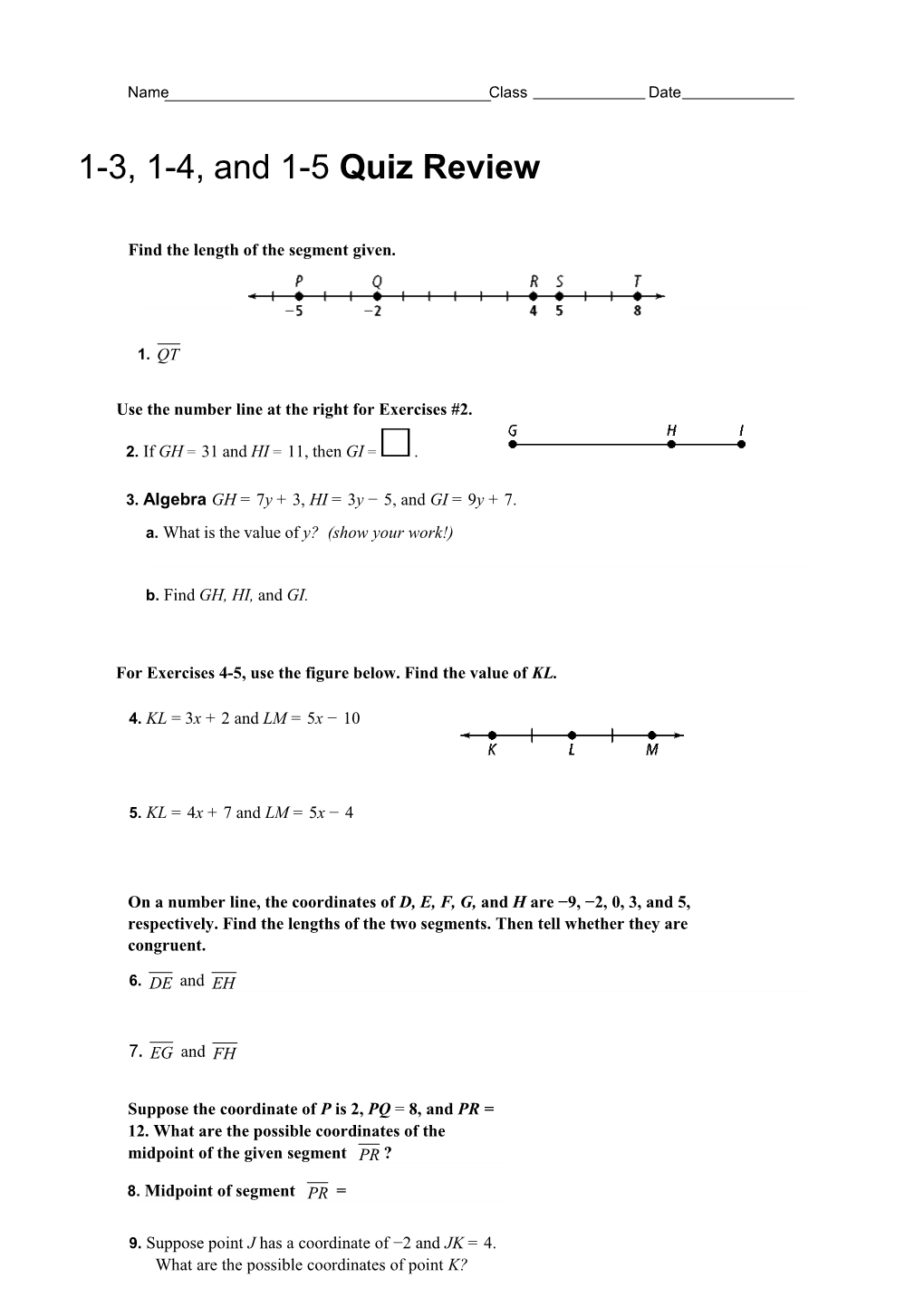# Use the Number Line at the Right for Exercises #2Name

Class

Date

1-3, 1-4, and 1-5Quiz Review

Find the length of the segment given.

1.

Use the number line at the right for Exercises #2.

2. IfGH=31andHI=11,thenGI=.

3. Algebra GH = 7y + 3, HI = 3y −5, and GI = 9y + 7.

b.FindGH,HI,andGI.

For Exercises 4-5, use the figure below. Find the value of KL.

4. KL = 3x + 2 and LM = 5x − 10

5. KL = 4x + 7 and LM = 5x − 4

On a number line, the coordinates of D, E, F, G, and H are −9, −2, 0, 3, and 5, respectively. Find the lengths of the two segments. Then tell whether they are congruent.

6. and

7. and

Suppose the coordinate of P is 2, PQ =8, and PR =12. What are the possible coordinates of the midpoint of the given segment ?

8.Midpoint of segment =

9. Suppose point J has a coordinate of −2 and JK = 4. What are the possible coordinates of point K?

Algebra Use the diagram at the right for Exercises 10-12.

10. IfNO=17andNP=5x−6,findthevalueofx. Then find NP and OP.

11. IfNO=3x+4andNP=10x−10,findthevalueofx. Then find NO, NP, and OP.

12. VocabularyWhat term describes the relationship between and

Name the shaded angle in three different ways. To start, identify the rays that form each angle.

13.

Use the diagram below. Find the measure of each angle. Then classify the angle as acute, right, obtuse, or straight.

14.AFB

To start, identify AFB. Then use the definition of the measure of an angle to find mAFB.

mAFB =

This angle is a(n) angle.15.CFD

16.AFE

17.AFC

Use the diagram at the right. Complete each statement.

18. PMJ

19. IfmLMP=100,thenmQHG= .

20. If mFHI = 142, what are mFHG and mGHI?

21. JKL is a right angle. What are mJKM and mMKL?

Algebra Use the diagram at the right for Exercise 22. Solve for x. Find the angle measures to check your work.

22.mCGD = 2x − 2, mEGF = 37, mCGF = 7x + 2

Use the diagram at the right. Is each statement true? Explain.

23. 6 and 5 are adjacent angles.

24. 1 and 2 are a linear pair.

Name an angle or angles in the diagram described by each of the following.

25.a pair of vertical angles

26.supplementary to RPS

To start, remember that supplementary angles are two angles whose measures have a sum of

27.apairofcomplementaryangles

To start, remember that complementary angles are two angles whose measures have a sum of.

For Exercises 8–11, can you make each conclusion from the information in the diagram? Explain.

29. CEGFED30.

Use the diagram at the right for Exercises 33 and 34.

33. Nametwopairsofanglesthatformalinearpair.

34. Nametwopairsofanglesthatarecomplementary.

35.Algebra In the diagram, bisects WXZ.

a.Solve for x and find mWXY.

b.Find mYXZ.

c.Find mWXZ.

Algebra bisects PQS. Solve for x and find mPQS.

36.mPQR = 3x, mRQS = 4x − 9

37.mPQR = 5x − 4, mSQR = 3x + 10